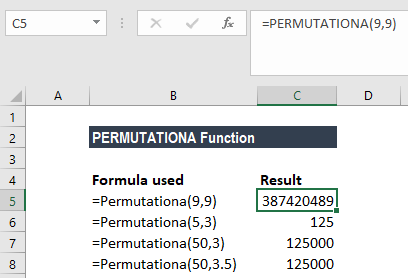# PERMUTATIONA Function

Returns the number of possible permutations for a specified number of objects

## What is the PERMUTATIONA Function?

The PERMUTATIONA Function is an Excel Statistical function. It returns the number of permutations that are possible for a specified number of objects in a given set. Introduced in MS Excel 2013, it’s important to note the function includes repetitions in the number it returns.

### Formula

=PERMUTATIONA(number, number_chosen)

The PERMUTATIONA function includes the following arguments:

1. Number (required argument) – This is an integer that describes the total number of objects.
2. Number_chosen (required argument) – An integer that describes the number of objects in each permutation.

### How to use the PERMUTATIONA Function in Excel?

To understand the uses of the function, let’s consider a few examples:

We wish to calculate the number of permutations (with repetitions) of six objects, selected from different sized sets.As you can see above, the function truncates the decimal value to integers, so we got the same result for:

PERMUTATIONA(50,3) and PERMUTATIONA(50,3.5), that is 125,000 possible permutations.

Combinations and permutations are different and, hence, they use different formulas. In a combination, the objects can be in any order, whereas in a permutation, the order is important.

### Difference between PERMUT and PERMUTATIONA Functions

Both the PERMUT and PERMUTATIONA functions calculate the number of permutations of a selection of objects from a set.

However, there is a difference between the two functions. PERMUT does not count repetitions whereas PERMUTATIONA counts repetitions.

For example, in a set of three objects –  a, b, and c – how many permutations are possible?

Under the PERMUT function, the result will give six possible permutations:

1. ab
2. ba
3. ac
4. bc
5. ca
6. cb

Whereas, the PERMUTATIONA function will return the following results:

1. aa
2. ab
3. ac
4. ba
5. bb
6. bc
7. ca
8. cb
9. cc

### A few notes about PERMUTATIONA Function

1. #VALUE! error – Occurs when “number” or “number_chosen” is not numeric.
2. #NUM! error – Occurs when:
1. The supplied number argument less than 0; or
2. The supplied number_chosen argument is less than 0; or
3. The supplied number argument is less than the number_chosen argument.

Thanks for reading CFI’s guide to important Excel functions! By taking the time to learn and master these functions, you’ll significantly speed up your financial analysis. To learn more, check out these additional CFI resources:

• Excel Functions for Finance